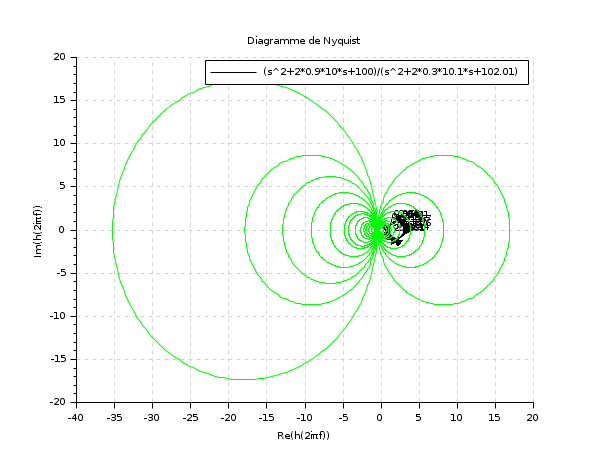Scilab Home page | Wiki | Bug tracker | Forge | Mailing list archives | ATOMS | File exchange
Change language to: English - Português - 日本語 - Русский

# m_circle

plots the complex plane iso-gain contours of y/(1+y) (obsolete)

### Calling Sequence

```m_circle()
m_circle(gain)```

### Arguments

gain

vector of gains (in DB). The default value is

gain

=[-12 -8 -6 -5 -4 -3 -2 -1.4 -1 -.5 0.25 0.5 0.7 1 1.4 2 2.3 3 4 5 6 8 12]

### Description

`m_circle` draws the iso-gain contours given by then `gain` argument in the complex plane (Re,Im).

The default value for `gain` is:

`[-12 -8 -6 -5 -4 -3 -2 -1.4 -1 -.5 0.25 0.5 0.7 1 1.4 2 2.3 3 4 5 6 8 12]`

`m_circle` is used with `nyquist`.

This function is superseded by the hallchart function.

### Examples

```s=poly(0,'s')
h=syslin('c',(s^2+2*0.9*10*s+100)/(s^2+2*0.3*10.1*s+102.01))
nyquist(h,0.01,100,'(s^2+2*0.9*10*s+100)/(s^2+2*0.3*10.1*s+102.01)')
m_circle();``````clf();
s=poly(0,'s')
h=syslin('c',(s^2+2*0.9*10*s+100)/(s^2+2*0.3*10.1*s+102.01))
h1=h*syslin('c',(s^2+2*0.1*15.1*s+228.01)/(s^2+2*0.9*15*s+225))
nyquist([h1;h],0.01,100,['h1';'h'])
m_circle([-8 -6 -4]);```